﻿ Text Encryption Based on Glider in the Game of LifeInternational Journal of Information Science

p-ISSN: 2163-1921    e-ISSN: 2163-193X

2016;  6(1): 20-27

doi:10.5923/j.ijis.20160601.02### Text Encryption Based on Glider in the Game of Life

Majid Vafaei Jahan, Faezeh Khosrojerdi

 Email: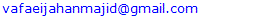Abstract

The Game of Life cellular automaton is a classic example of a massively parallel collision-based computing device. The automaton exhibits mobile patterns, gliders, and generators of the mobile patterns, glider guns, in its evolution. We show how to construct basic logical operations, AND, OR, NOT in space-time configurations of the cellular automaton. Also, decomposition of complicated Boolean functions is discussed. Also in this paper advantages of our technique are demonstrated on an example of a method for text encryption by mapping text to binary space. This method is based on glider and rules of Game of Life.

Keywords: Cellular Automata, Text encryption, Glider, Game of Life

Cite this paper: Majid Vafaei Jahan, Faezeh Khosrojerdi, Text Encryption Based on Glider in the Game of Life, International Journal of Information Science, Vol. 6 No. 1, 2016, pp. 20-27. doi: 10.5923/j.ijis.20160601.02.

### 1. Introduction

Cellular automata are mathematical models for complex natural systems containing large numbers of simple identical components with local interactions. They consist of a lattice of sites, each with a finite set of possible values. The values of the sites evolve synchronously in discrete time steps according to identical rules. The value of a particular site is determined by the previous values of a neighborhood of sites around it [10, 22]. There is a classification for CA that comparing their behavior with that of some continuous dynamic systems . Wolfram specifies four classes of CA on the basis of qualitative criteria. For all initial configurations, Class 1 automata evolve after a finite time to a homogeneous state where each cell has the same value. Class 2 automata generate simple structures where some stable or periodic forms survive. Class 3 automata’s evolution leads, for most initial states, to chaotic forms. All other automata belong to Class 4 [5, 6]. Among two-dimensional and two-positions members of the class 4, Game of Life automata looks like a good candidate for modeling the behavior of complex systems. Conway proved its ability to simulate a Turing machine, using gliders and glider guns [6, 10]. Gliders are mobile self-localized patterns of non-resting states, and glider guns are patterns which when evolving alone, periodically recover their original shape after emitting some gliders. Glider gun emits a glider stream that carries information and can create logic gates and logical function through collisions [5, 6].
In this paper with a brief introduction of CA and Game of Life, glider, and its characteristics as one of the patterns of Game of Life are described. After that, the implementation of logical gates and logical functions has been studied using this pattern. Finally, a method of text encryption is introduced, this method is based on the features glider and logical functions are implemented by them and it can be considered as one of the glider applications.

### 2. Cellular Automata

A cellular automaton is a discrete model. It consists of a regular grid of cells, each in one of a finite number of states, such as "0" and "1". The grid can be in any finite number of dimensions. At this paper two dimensional CA are used.
A 2- CA (2 dimensional cellular automata) can be defined as a 4-tuple . Expression (1) shows this tuple.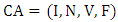(1)
Where I is the cellular space formed by a two-dimensional array of r×c cells that is shown in expression (2):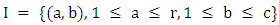(2)
Let I denote the set of integer, I is a set of Cartesian product of two integer sets , V is a set of cellular states ,N is the type of neighborhood, and f is the local transition function from Vn to V . The neighborhood of a cell (a, b) is the set of cells whose states at time t determine the state of the cell (a, b) at time t + 1, by means of the local transition function. Among the variety of existing neighborhoods, two common neighborhoods with different radius are shown in Figure (1).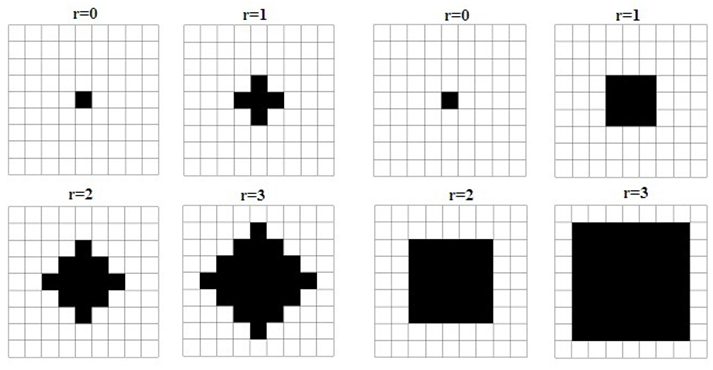Figure (1). CA neighborhood Von Neumann neighborhood moore neighborhood

### 3. Game of Life

In 1970, Conway discovered a special cellular automaton (that he called the Game of Life) that was later popularized by Gardner [6, 26]. The Game of Life is a two dimensional cellular automaton with binary cell states and Moore neighborhood. Each cell of the automaton takes either 0 or 1 state (can be called them living or dead) and updates its state in discrete time depending on the states of its eight closest neighbors and the four simple rules:
1. Any live cell with fewer than two live neighbors dies.
2. Any live cell with two or three live neighbors, lives on to the next generation.
3. Any live cell with more than three live neighbors dies.
4. Any dead cell with exactly three live neighbors becomes a live cells [6, 12].
The initial pattern as well as the rules of game generates next populations of game by births and mortalities in the lattice.

#### 3.1. Game of Life Patterns

It is possible to fill a lattice with 0s and 1s at random and allow the configurations to develop for a while find that after some time most patterns inhabiting the lattice can be classified as follows:
1. Stable pattern: They remain in unchanged if not disturbed.
2. Repeating pattern: They usually change their configuration in a very short cycle. These patterns can be mobile or immobile in lattice .
Some examples of these two classes are shown in figure (2) [2, 18].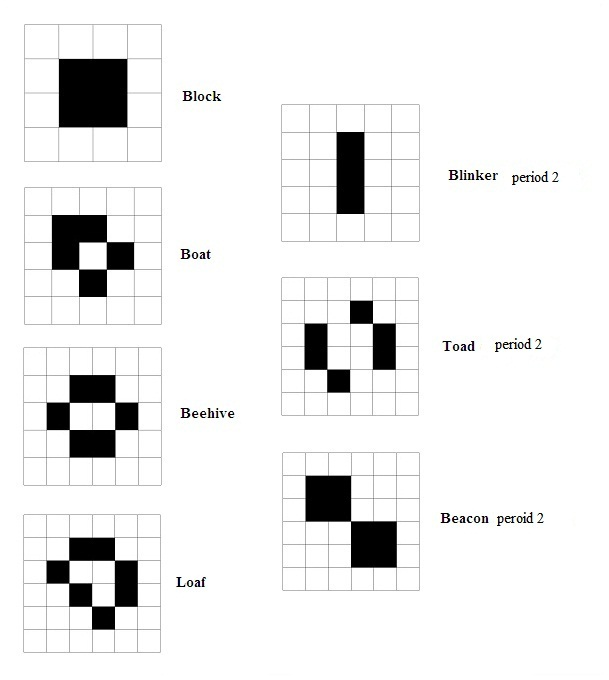Figure (2). Some examples of Game of Life pattern

### 4. Glider

The glider is a repeating pattern that travels across the lattice in Conway's Game of Life. Their movement is diagonal in cellular lattice  and after one period come back to initial pattern and this procedure continues [12, 15]. Therefore important feature of gliders is their movement in cellular lattice. Figure (3) shows a glider whit period 4 . This glider is used in this paper.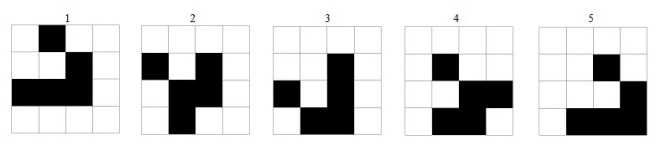Figure (3). Glider

#### 4.1. Glider Gun

In a cellular automaton, a glider gun is a pattern with a main part that repeats periodically and that also periodically emits gliders . A glider gun is localized pattern that periodically lose its stability and give birth to traveling mobile localization, gliders . There are then two periods that may be considered: the period of the spaceship output, and the period of the gun itself, which is necessarily a multiple of the spaceship output's period . A glider gun generates a glider in any period . After some periods a stream of gliders is emitted in cellular lattice and this stream is mobile and the distance between both gliders is the same. This distance depends on the glider guns period. The shorter period of the gun, the less distance. Figure (4) shows a glider gun for the glider is shown figure (3). This gun emits a glider every 30th set of discrete time (period=30) [2, 17].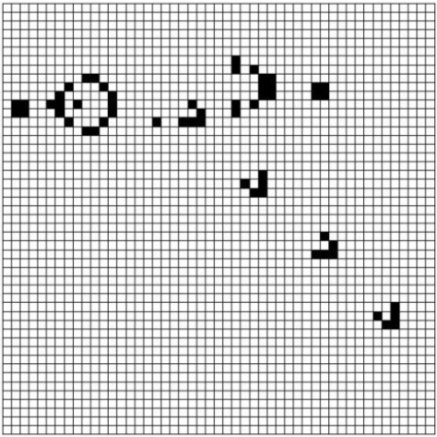Figure (4). Glider gun
Discovery algorithms of glider guns are based on genetic algorithm [4, 5, 6, 23] that are out of this paper.

#### 4.2. Glider Collision

A gun that emits gliders rightward is named right-gun and a gun that emits gliders leftward is named left-gun . If a right-gun and a left-gun are placed side by side, their streams of glider will cancel each other as a result of glider collisions .
When gliders are at the same vertically level when they collide with each other, the process of glider streams annihilation is two-phase. Firstly, collision of two gliders generates two 2*2 blocks. The gliders following the two previous ones crash into the blocks. The blocks and gliders disappear as result of the collision [2, 8]. This process takes a period of 27.
For simplify and reduce the time, and also given that the application is considered in this paper, the simulation can be used for this collision.
According to figure (5) collision between a glider and a block will cause the loss of both of them. The same feature can be used to simulate the collision among gliders. When two gliders place in position 23 of Figure (5), collisions among them can be predicted and with put the two blocks in the path, this collision is simulated. In this condition, number of steps to eliminate these two gliders is reduced to 5 steps. In this paper simulated collision will be used.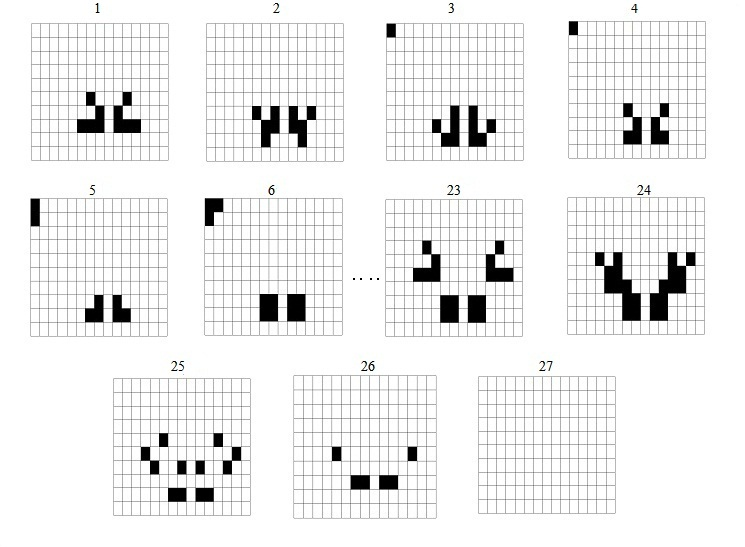Figure (5). Glider collision

#### 4.3. Eater

Ideal eaters are periodic patterns that, after the absorption of a glider, can resume their original shape and position quickly enough to absorb another arriving glider . Figure (6) shows an eater and collision with the glider. In this paper that eater will be used.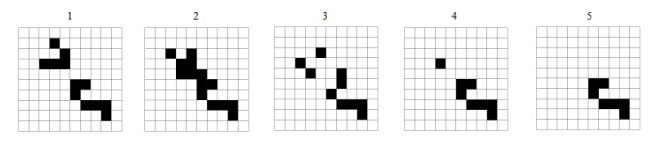Figure (6). A eater that consume the glider

### 5. Implementation of Logical Gates

Logic gates are binary operators with binary input and output. Important logical gates are AND, OR and NOT. These gates are shown in Table (1), A and B are gate inputs.
 Table (1). AND, OR, NOT gates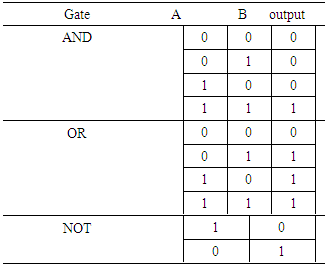There are three main points in Implementation of logic gates:
Ÿ Some kind of electrical pulses to represent inputs.
Ÿ Wires to transmit the electrical pulses.
Ÿ Processing devices which associate inputs and compute the Boolean result.
To implement logical gates using gliders, we thus encode these items in the Game of Life objects as follows:
Ÿ Input and output electrical pulses >> gliders.
Ÿ Wires >> Trajectories of glider movements.
Ÿ Processing devices >> collision of gliders .
Given the above mapping, for each input pulse, a glider gun is used. Therefore glider stream that emitted by the gun specifies the input pulse. These guns are named input guns. The glider stream path represents the wire carrier electric pulses and collision among gliders is used to determine the output of gates.
Depending on the gate, some glider guns is considered to implement collision. These guns have no role in producing input, but they emit a fixed glider stream for implement collision. These gliders are named process guns. To destroy some of the gliders which have no role in determining the output, some eaters are used.
We must represent electrical pulses going along input wires. The pulses can be implemented with a glider gun that generates a stream of gliders [2, 21]. When an input gun emits a glider, an input pulse is determined for gate. Generally speaking, a stream of gliders can encode any data. For example, the number "101" will be a series "glider - no glider - glider". We also want to control glider stream according to the data they carry. Like in an electrical circuit, the gliders must propagate only if the input is true. We must therefore find a way to stop the gliders if the input is false [2, 4].
This can be done via coupling the glider gun with a block. As we have seen in the previous sections the block eliminates a glider in a collision.
Input gun produces an electrical pulse in each period. If the input pulse is true it would indicate with produced glider at that period and this glider moves in cellular automaton along its path. But if the input pulse is false, produced glider in the period should be eliminated by a block. This block should be placed in its path immediately after glider production. Therefore in input stream, a glider indicates a true pulse and a missing glider indicates a false pulse.
For the gate s output, a cell with the following conditions is considered in the lattice:
Ÿ It should be along the gliders path that will determine the output.
Ÿ It should be lower than the last collision that may be on cellular lattice.
Therefore, to obtain the output value we simply have to check a state of one specific cell. If the cell is activated the output value will be considered to be true, otherwise it will be false. The first output is determined after the time that needs to reach the first input into the output cell and for another outputs, this cell should be checked at period equivalent guns period.
According this description, Table (2) shows needs to implement the basic gates:
 Table (2). Needs to implement the basic gates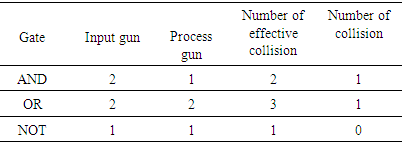Implementation of three basic gates, AND, OR and NOT are shown in figures (7), (8) and (9).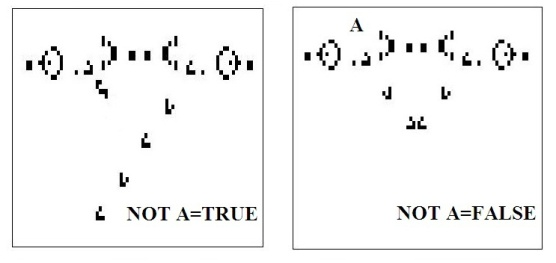Figure (7). Configurations of the automaton for various input values of the NOT-gate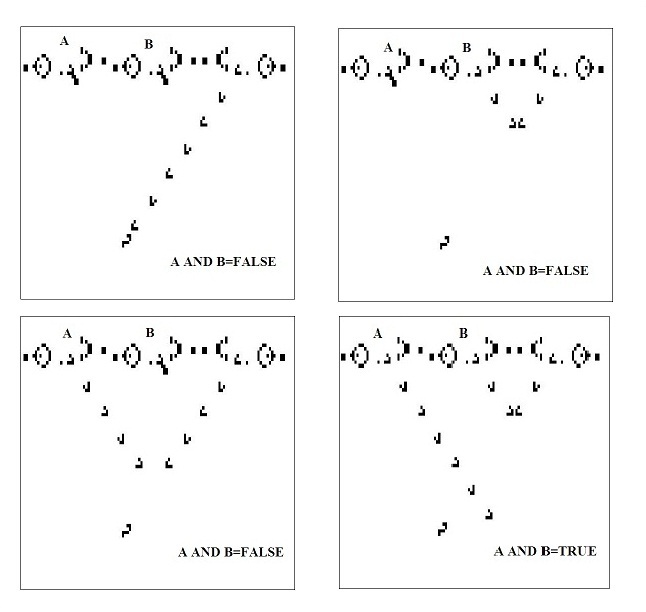Figure (8). Configurations of automaton for various input values of the AND-gate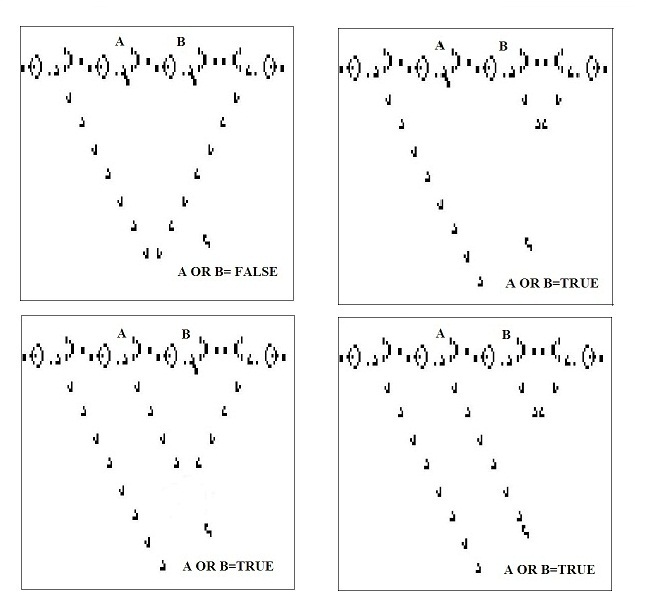Figure (9). Configurations of the automaton for various input values of the OR-gate

### 6. Implementation of Other Logical Functions

Using the proposed gates, we can implement another logical gates and functions. In these implementations number of process guns should be considered. If number of the gun be less, the number of collisions would have been less and the output would have generated faster. Thus implementation of the logic function, using three main gates, should have minimum necessary gun.

#### 6.1. XOR Implementation

The XOR operator is shown in Table (3). There are some combinational logic functions for this gate. To implement this gate using gliders in CA, we should find a logical function that needs lowest guns. For example, we compare expression (2) and expression (3) .
 Table (3). XOR operation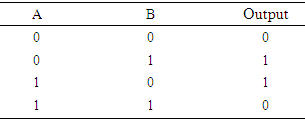At the first in expression (2) according to the precedence of operators, we should implement expression (A AND (NOT B) ). According to Table (2) this implementation requires tow input guns and two process guns. Also implementation of expression ((NOT A)AND B) requires two input guns and two process guns. Finally implementation of middle OR gate requires two process guns. Generally implementation of expression (2) requires ten guns.
At the first in equation (3) according to the precedence of operators, we should implement expression ((NOT A)AND B). According to Table (2) this implementation requires tow input guns and two process guns. Also implementation of expression (AOR B) requires two input guns and two process guns. Finally implementation of middle AND gate requires one process gun. Generally implementation of expression (3) requires nine guns. Therefore expression (3) is more appropriate for implementation of XOR gate. This implementation is shown in figure (10).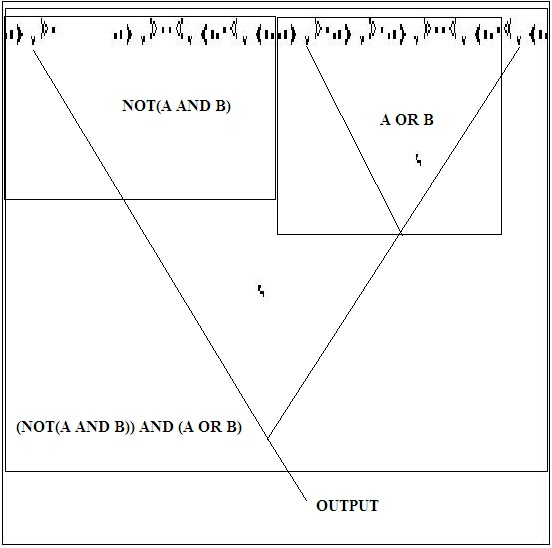Figure (10). Implementation of XOR gate
In this figure we can see greater distance between first and second gun because gliders of first and latest gun should reach the collision point at a same time.

### 7. Text Encryption

In the previous sections the glider concepts and implementation of logic functions were described. In this section a text encryption method is described that is based on the glider concepts in the Game of Life.

#### 7.1. Text-to-Glider

The first step is to convert text to the glider stream. According to unique nature of ASCII code for all characters, any character can be indicated by 7 binary bits. This binary string is equivalent to character s ASCII code. Now this string can be converted to glider steam. This conversion is done by considering the following two rules:
Ÿ In binary string, 1 is equal to one glider in glider stream.
Ÿ In binary string, 0 is equal to a missing glider in glider stream.
The same procedure can be converted the text to the glider stream.
For example conversion of “glider” to its equivalent glider stream is shown in Table (4).
 Table (4). Conversion of “glider” to its equivalent glider stream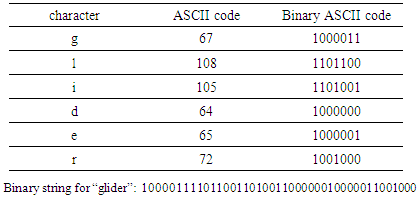We should generate this glider stream by input glider gun. For that we use the equivalent binary string of the plain text. In each period, if the input bit is 1, it would indicate by produced glider at that period but if the input bit is 0, produced glider in that period should be eliminated by a block.
The equivalent binary string of ASCII code for each character is 7 bits therefore the equivalent glider stream length is 7 gliders. To reduce this length we can consider only the characters that used in the plain text and table made a similar table to ASCII table. Thus the length of equivalent binary string and the length of equivalent glider stream for each character will be shorter.

#### 7.2. Select the Encryption Key

In the second step, a proper key should be selected for the encryption. This key can be generated randomly by a random number generator. Then the key must be converted to corresponding glider stream. For this conversion we should convert binary key to corresponding glider stream. Also the key can be a string of characters that should be converted to glider stream. This conversion is similar to conversion of plain text to glider stream. If the length of key is shorter than the length of text, repeat of the key can be used.

#### 7.3. Cryptographic Operation

Two glider streams produced in sections 7-2 and 7-3 are used as inputs XOR gate design in figure (10). Output will be as a stream of gliders that can convert to encrypted text and method of this conversion is the reverse conversion method of plain text to glider stream.
For decoding, this process is repeated but one input of XOR gate will be corresponding glider stream of encrypted text.

### 8. Conclusions

This paper reviews the features of glider in the Game of Life CA. It showed how to simulate the basic logical gates based on CA and Game of Life rules. The main point in this simulation is feature of gliders and their moving in CA. We can also combine these basic gates to implement other logical gates and functions in CA. By expanding on the multi-bits input for the gates, implementation of binary calculations will be possible in CA. Emitted gliders can be controlled by eater and block and this help us to mapping text to glider. Therefore implementation of non binary discussion is possible in CA.
The glider stream can contain a text. We can encrypt this text by features of glider. Encryption function is XOR gate and encryption key is generated randomly. Also decryption function is XOR gate and it implement by glider. So this method is based on only three rules of Game of Life.

### References

  Hodjat, S, Meybodi, M. R., "Adaptation of Q-Learning Parameters using Games of Learning Automata", Proceedings of the 3th Iranian Annual Computer Conference, CSICC'97, pp. 33-48, Science and Technology University, Tehran, Iran, December 23-25, 1997.  Jean-Phiippe Rannard, “Implementation of logical functions in the Game of Life” In A. Adamatzky(Ed), collision-based computing, pp. 491-512, London, 2002.  Emannuel Sapin, L. Ball, A. Adamatzky, “Genetic approaches to search for computing pattern in cellular automata”, IEEE computational intelligence magazine, university of England, UK, August 2009.  Emmanuel Sapin, Larry Bull, “Evolutionary search for cellular automata logic gates with collision based computing”, Faculty of computing, engineering and mathematical science, university of the west of England, complex systems, 2008.  Emmanuel sapin, Olivier Bailleux, Jean-Jacques Chabrier, “Research of a cellular automaton simulating logic gates by evolutionary algorithms”, university Bourgogne, LERSIA, 2008.  Emmanuel Sapin, Olivier Bailleux, Jean-Jacques Chabrier, “Research of complex form in the cellular automata by evolutionary algorithms”, P.liardet et al. (Eds.): EA 2003, LNCS 2936, pp. 357-367, University Bourgogne, 2004.  E.spain, O. Bailleux, J-J. Chabrier, P. Collet, “Demonstration of the universality of a new cellular automata”, International Journal of Unconventioned Computing, vol.3, pp.79-103, University Bourgogne, 2007.  Emmanuel sapin, Olivier Bailleux, Jean-Jacques Chabrier, “A new approach of stream duplication in 2D cellular automata”, In GECCO04, Lecture Notes in Computer Science, 3102, pp. 175-187, university Bourgogne , 2004.  E.spain, L. Bull, “Searching for glider guns in cellular automata: exploring evolutionary and other techniques”, Lecture Notes in computer Science, university of the west of England, 2008.  S. Wolfram, “Universality and complexity in cellular automata”, In Physica D: Nonlinear Phenomena, vol. 10, pp.1-35, 1984.  Kumar, Tapas, Sahoo, G, “A Novel Method of Edge Detection using Cellular Automata”, International Journal of Computer Application, Vol.9-No.4, pp. 38-44, November 2010.  Gardner, Martin, Mathematical Games, The fantastic combinations of John Conway's new solitaire game life, pp. 120–123. ISBN 0894540017. Archived from the original on 2009-06-03.  Paul Chapman, Life Universal Computer, Retrieved July 12, 2009, http://www.igblan.freeonline.co.uk/ igblan/ca/.  E.spain, L. Bull,” the emergence of glider guns in cellular automata found by evolutionary algorithms”, engineering and mathematical science, university of the west of England, 2008.  Stephen A. Silver, Glider, The Life Lexicon, Retrieved July 12, 2009.  Jason Summers, Game of Life Status page, retrieved 2012-02-23.  Stephen A. Silver, Gosper glider gun, The Life Lexicon.  Andrzej Okrasinski, Game of Life Object Statistics, Archived from the original on 2009-07-27.  Genaro J. Martne, z Andrew Adamatzky, Harold V. McIntosh, “Localization dynamics in a binary two-dimensional cellular automaton: the Diffusion Rule”, submitted to Journal of Cellular Automata, submitted August 6, 2006 accepted December 2006.  Berlekamp E.R., Conway J.H. & Guy R. Winning Ways for Your Mathematical Plays, vol 2 (Academic Press, 1982). The structures of our basic gates are introduced in this text.  Jakubowski, M.H., Steiglitz, K., & Squier, R. (2001) Computing with Soli- tons: “A Review and Prospectus, Multiple-Valued Logic, Special Issue on Collision-Based Computing” 6 (5-6).  Gri_eath, D. & Moore, C. (Eds.) (2003) “New constructions in cellular au-tomata”, (Santa Fe Institute Studies on the Sciences of Complexity) Oxford University Press.  Das, R., Mitchell, M. & Crutch_eld, J.P. (1994) “A genetic algorithm dis-covers particle-based computation in cellular automata”, Lecture Notes in Computer Science 866 34-353.  S. Wolfram, N.H. Packard, “Two-dimensional cellular automata”, Journal of Statistical Physics, 38:901-946, 1985.  Adamatzky, A. (Ed.) Collision-Based Computing, Springer, 2003.  Heudin, J.-K., “A new candidate rule for the game of two-dimensional life”, Complex Systems, 1996, 10 367-381.  McIntosh, H.V. Wolfram's Class IV and a Good Life, Physica, 1990, D 45 105-121.